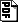## Nondeterministic Circuit Lower Bounds from Mildly Derandomizing Arthur-Merlin Games#### Abstract

In several settings derandomization is known to follow from circuit lower bounds that themselves are equivalent to the existence of pseudorandom generators. This leaves open the question whether derandomization implies the circuit lower bounds that are known to imply it, i.e., whether the ability to derandomize in any way implies the ability to do so in the canonical way through pseudorandom generators.

For the setting of decision problems, Impagliazzo et al. implicitly showed the following equivalence: Randomized polynomial-time decision procedures can be simulated in NSUBEXP (the subexponential version of NP) with subpolynomial advice on infinitely many input lengths if and only if NEXP is not in P/poly. We establish a full analogue in the setting of verification procedures: Arthur-Merlin games can be simulated in Σ2SUBEXP (the subexponential version of Σ2P) with subpolynomial advice on infinitely many input lengths if and only if Σ2EXP is not in NP/poly.

A key ingredient in our proofs is improved Karp-Lipton style collapse results for nondeterministic circuits. The following are two instantiations that may be of independent interest: Assuming that Arthur-Merlin games can be derandomized in Σ2P, we show that

• if PSPACE is contained in NP/poly then PSPACE collapses to Σ2P, and
• if coNP is contained in NP/poly then PH collapses to P^Σ2P.

dieter@cs.wisc.edu